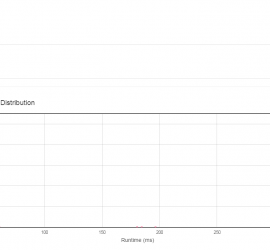# Daily Archives: August 9, 2015

## [leetcode] Power of Two

Power of Two Given an integer, write a function to determine if it is a power of two. //can n be negative? //negative number is definitely not a power of two //1 is power of 2 //0 is not //1 2 4 8 16 32 64 .. class Solution { […]## [leetcode] Max Points on a Line

Max Points on a Line Given n points on a 2D plane, find the maximum number of points that lie on the same straight line. First, I thinked out an o(n^3) force solution. It’s workable at last. However, an o(n^2) solution exists which uses a hash table to store the slope […]